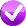# Building Java Programs, 3rd edition

## Lab: Return

Except where otherwise noted, the contents of this document are Copyright 2013 Stuart Reges and Marty Stepp.

lab document created by Marty Stepp, Stuart Reges and Whitaker Brand

# Lab goals

Goals for this problem set:

• use `return` values to send data between methods

# Returning Values

A return value is when a method sends a value back to the code that called it.

```public static type name(parameters) {      // declare
...
return expression;
}
```
```variableName = methodName(parameters);     // call
```

Example:

```public static double fToC(double tempF) {
return (tempF - 32) * 5.0 / 9.0;
}
...
double bodyTemp = fToC(98.6);          // bodyTemp stores 37.0
double freezing = fToC(32);            // freezing stores  0.0
```

# Math expression syntax

Method Description Example
`Math.abs` absolute value `Math.abs(-308)` returns `308`
`Math.ceil` ceiling (rounds upward) `Math.ceil(2.13)` returns `3.0`
`Math.floor` floor (rounds downward) `Math.floor(2.93)` returns `2.0`
`Math.max` max of two values `Math.max(45, 207)` returns `207`
`Math.min` min of two values `Math.min(3.8, 2.75)` returns `2.75`
`Math.pow` power `Math.pow(3, 4)` returns `81.0`
`Math.round` round to nearest integer `Math.round(2.718)` returns `3`
`Math.sqrt` square root `Math.sqrt(81)` returns `9.0`

# Exercise - Math expressions

Write the results of each expression. Use the proper type (such as `.0` for a `double`). Note that a variable's value changes only if you re-assign it using the `=` operator. Discuss any errors you make with your neighbor.

```double grade = 2.7;

double min = Math.min(grade, Math.floor(2.9));   //   min = 2.0

double x = Math.pow(2, 4);                       //     x = 16.0
x = Math.sqrt(64);                               //     x = 8.0

int count = 25;
Math.sqrt(count);                                // count = 25
count = (int) Math.sqrt(count);                  // count = 5

int a = Math.abs(Math.min(-1, -3));              //     a = 3
```

# Exercise : areaConsider the following method for converting milliseconds into days:

```// converts milliseconds to days
public static double toDays(double millis) {
return millis / 1000.0 / 60.0 / 60.0 / 24.0;
}
```

Write a similar method named `area` that takes as a parameter the radius of a circle and that returns the area of the circle. For example, the call `area(2.0)` should return `12.566370614359172`. Recall that area can be computed as π times the radius squared and that Java has a constant called `Math.PI`.

• (Try solving this problem in Practice-It by clicking the icon above.)

# Exercise : payWrite a method named `pay` that accepts two parameters: a real number for a TA's salary, and an integer for the number of hours the TA worked this week. The method should return how much money to pay the TA. For example, the call `pay(5.50, 6)` should return `33.0`.

The TA should receive "overtime" pay of 1 ½ normal salary for any hours above 8. For example, the call `pay(4.00, 11)` should return (4.00 * 8) + (6.00 * 3) or `50.0`.

• (Try solving this problem in Practice-It by clicking the icon above.)